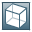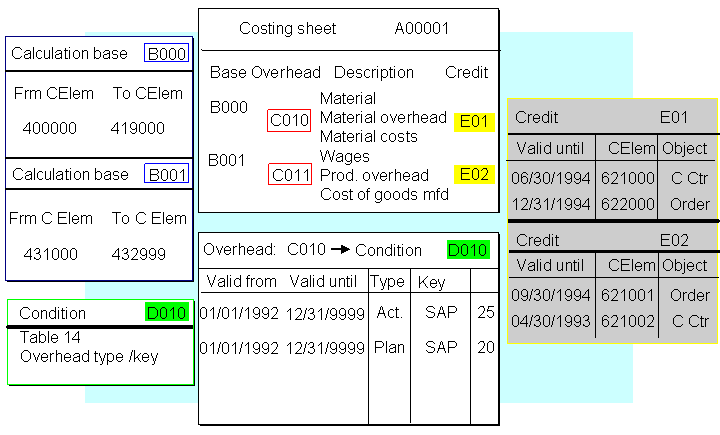Show TOCCosting SheetContains control parameters for all aspects of overhead calculation

You define the following parameters in a costing sheet:

• The direct costs to which overhead is applied (calculation base)

• The conditions under which overhead is applied (dependency)

• Whether overhead is allocated as a percentage or based on the quantity

• The overhead percentage rate, or the quantity-based overhead rate per unit of measure

• The validity period for the overhead rate

• Which object is credited (cost center, process, or order), and which cost element is used for actual credit postings (credit key)

If you are using Activity-Based Costing (CO-OM-ABC), the costing sheet also controls how process costs are allocated. For more information about the allocation of process costs, see Process Costs.

In material costing, you specify the costing sheet in the valuation variant in Customizing.

In Reference and Simulation Costing, you specify the costing sheet in the master record of the base planning object.

Structure

The costing sheet contains the following elements:

• Calculation base

The calculation base consists of a group of cost elements to which overhead is applied according to the same conditions. This process involves assigning individual cost elements or cost element intervals to a calculation base. This assignment is done separately for each controlling area.

You can apply different overhead amounts to the fixed and variable portions of a given base cost element. You can also have different overhead rates for different materials. You do this by entering the origin groups in the material master records and then specifying these origin groups in the calculation bases.

Overhead rates are applied to the calculation base either as a lump sum per quantity unit or as a percentage of the direct costs. You also specify the validity period and the conditions under which the overhead should be calculated. The system calculates the overhead amount either as a percentage or based on the quantity.

The conditions under which overhead is to be applied are defined in condition tables. The standard condition table is linked to a controlling area, an overhead type (planned or actual), and to one other field of the object's master record (such as the plant, or overhead key). Hence the conditions for overhead calculation can relate to all the reference objects of an organizational unit, or to an overhead key.

These rows also contain a credit key. The credit key determines the cost element with which the overhead is applied, and which cost center, business process or order is credited. You can also specify which part of the overhead should be reported as fixed costs.

• Totals rows

These rows contain subtotals.